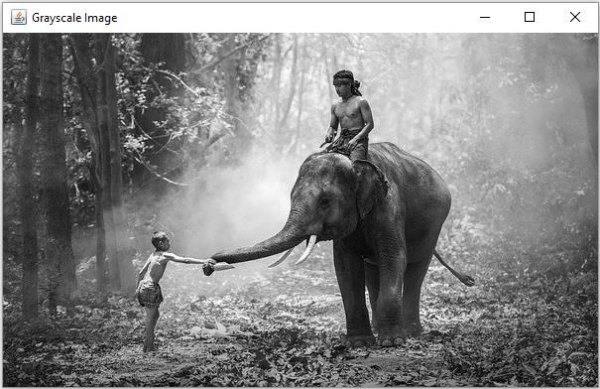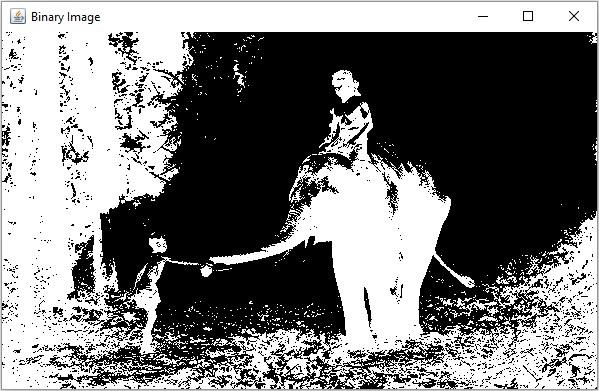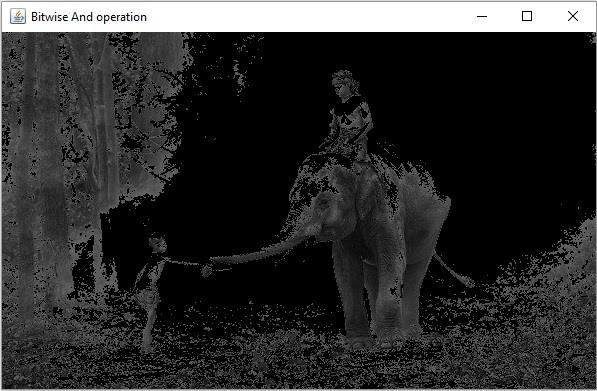# How to perform Bitwise And operation on two images using Java OpenCV?

JavaObject Oriented ProgrammingProgramming

You can compute bitwise conjunction between two images using the bitwise_and() method of the org.opencv.core.Core class.

This method accepts three Mat objects representing the source, destination and result matrices, calculates the bitwise conjunction of each every element in the source matrices and stores the result in the destination matrix.

## Example

In the following Java example we are converting an image into binary and gray scale and calculating the bitwise conjunction of the results.

import org.opencv.core.Core;
import org.opencv.core.Mat;
import org.opencv.highgui.HighGui;
import org.opencv.imgcodecs.Imgcodecs;
import org.opencv.imgproc.Imgproc;
public class BitwiseAndExample {
public static void main(String args[]) throws Exception {
String file ="D://images//elephant.jpg";
HighGui.imshow("Grayscale Image", src);
//Creating an empty matrix to store the results
Mat dst = new Mat(src.rows(), src.cols(), src.type());
Mat threshold = new Mat(src.rows(), src.cols(), src.type());
Mat gray = new Mat(src.rows(), src.cols(), src.type());
//Converting the gray scale image to binary image
Imgproc.threshold(src, threshold, 100, 255, Imgproc.THRESH_BINARY_INV);
HighGui.imshow("Binary Image", threshold);
//Applying bitwise and operation
Core.bitwise_and(src, threshold, dst);
HighGui.imshow("Bitwise And operation", dst);
HighGui.waitKey();
}
}

## Input Image## Output

On executing, the above program generates the following windows −

Gray Scale ImageBinary ImageBitwise And